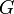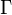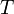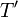# 15-344/Homework Assignment 5

This assignment is due at the tutorials on Thursday November 5. Here and everywhere, neatness counts!! You may be brilliant and you may mean just the right things, but if the teaching assistants will be having hard time deciphering your work they will give up and assume it is wrong.

Additional Problem. Given a connected graph$G$, let$\Gamma$ be the graph whose vertices are the spanning trees of$G$, and where two vertices$T$ and$T'$ in$\Gamma$ are connected by an edge in$\Gamma$ if$T'$ is obtained from$T$ by removing one edge and adding one edge. Prove that$\Gamma$ is itself a connected graph.
Answer. The vertices of a graph can be an arbitrary set. A set of apples, or of oranges, or of numbers, or of letters, or of spanning trees in another graph. In other words, for every spanning tree of the graph$G$, there's a vertex in the graph$\Gamma$. If$G$ has 125 spanning trees, say, then$\Gamma$ would have 125 vertices. --Drorbn (talk) 09:44, 3 November 2015 (EST)